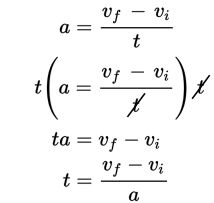# SAT Math Multiple Choice Question 320: Answer and Explanation

### Test Information

Question: 320

5.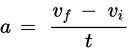Acceleration is the rate at which the velocity of an object changes with respect to time, or in other words, how much an object is speeding up or slowing down. The average acceleration of an object can be found using the formula shown above, where t is the time over which the acceleration is being measured, vf is the final velocity, and vi is the initial velocity. Which of the following represents t in terms of the other variables?

• A.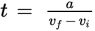• B.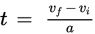• C. t = a(vf - vi)
• D.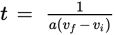Explanation:

B

Difficulty: Medium

Category: Passport to Advanced Math / Exponents

Strategic Advice: This question looks complicated, but really just amounts to solving the equation for t using a couple of inverse operations.

Getting to the Answer: Multiply both sides of the equation by t to get it out of the denominator, and then divide both sides by a.